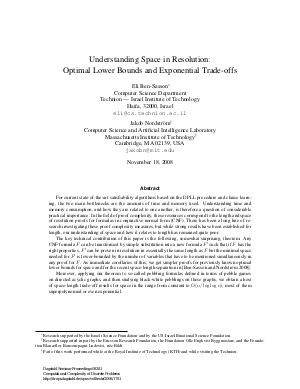Document# Understanding space in resolution: optimal lower bounds and exponential trade-offs

### Authors Eli Ben-Sasson, Jakob Nordström## File

DagSemProc.08381.6.pdf
• Filesize: 474 kB
• 49 pages

## Cite As

Eli Ben-Sasson and Jakob Nordström. Understanding space in resolution: optimal lower bounds and exponential trade-offs. In Computational Complexity of Discrete Problems. Dagstuhl Seminar Proceedings, Volume 8381, Schloss Dagstuhl - Leibniz-Zentrum für Informatik (2008)
https://doi.org/10.4230/DagSemProc.08381.6

## Abstract

We continue the study of tradeoffs between space and length of resolution proofs and focus on two new results: begin{enumerate} item We show that length and space in resolution are uncorrelated. This is proved by exhibiting families of CNF formulas of size \$O(n)\$ that have proofs of length \$O(n)\$ but require space \$Omega(n / log n)\$. Our separation is the strongest possible since any proof of length \$O(n)\$ can always be transformed into a proof in space \$O(n / log n)\$, and improves previous work reported in [Nordstr"{o}m 2006, Nordstr"{o}m and H{aa}stad 2008]. item We prove a number of trade-off results for space in the range from constant to \$O(n / log n)\$, most of them superpolynomial or even exponential. This is a dramatic improvement over previous results in [Ben-Sasson 2002, Hertel and Pitassi 2007, Nordstr"{o}m 2007]. end{enumerate} The key to our results is the following, somewhat surprising, theorem: Any CNF formula \$F\$ can be transformed by simple substitution transformation into a new formula \$F'\$ such that if \$F\$ has the right properties, \$F'\$ can be proven in resolution in essentially the same length as \$F\$ but the minimal space needed for \$F'\$ is lower-bounded by the number of variables that have to be mentioned simultaneously in any proof for \$F\$. Applying this theorem to so-called pebbling formulas defined in terms of pebble games over directed acyclic graphs and analyzing black-white pebbling on these graphs yields our results.
##### Keywords
• Proof complexity
• Resolution
• Pebbling.

## Metrics

• Access Statistics
• Total Accesses (updated on a weekly basis)
0